# SSC Quantitative Aptitude Questions (Day-44)

Dear Aspirants, you can find the Quantitative Aptitude questions with detailed explanations for the SSC exams. Nowadays the competitive level of the exam has been increasing consistently. Due to the great demand for the government job, the level of the toughness reached greater. Candidates have to enhance the preparation process in order to drive in the right path. It doesn’t need to clear the prescribed cutoff. You must have to score good marks more than the cut off marks to get into the final provisional list. Here we have updating the Quantitative Aptitude questions with detailed explanations on a daily basis. You can practice with us and measure your level of preparation. According to that you can sculpt yourself in a proper way. SSC aspirants kindly make use of it and grab your success in your career.

Start Quiz

1)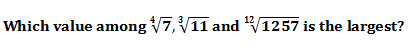(a) ∛11

(b) ∜7

(c) 12√(1257)

(d) All are equal

2)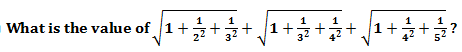(a) 18/5

(b) 4/3

(c) 7/3

(d) 33/10

3) What is the value of (203 + 107)2 – (203 – 107)2?

(a) 85886

(b) 86884

(c) 43442

(d) 87884

4) If 46N is divisible by 18, then what is the value of N?

(a) 2

(b) 4

(c) 7

(d) 8

5) Which is the smallest four digit number that is a perfect square?

(a) 1024

(b) 1048

(c) 1021

(d) 1089

6) Aman and Raman together complete a piece of work in 30 days, Raman and Manan can complete the same work in 36 days and Manan and Aman can complete the same work in 45 days. All of the three working together can complete the work in how many days?

(a) 12

(b) 18

(c) 24

(d) 28

7) Two pipes A and B can fill a tank in 20 hours and 24 hours respectively. If the two pipes opened at 5 in the morning, then at what time the pipe A should be closed to completely fill the tank exactly at 5 in the evening?

(a) 3 pm

(b) 2 pm

(c) 1 pm

(d) 11 am

8) The marked price of a cycle is Rs 36800. If 24% discount is given, then what will be the selling price (in Rs) of the cycle?

(a) 26168

(b) 27168

(c) 27968

(d) 28142

9) An article having marked price Rs 1800 is sold for Rs 1476. What is the discount percentage?

(a) 14

(b) 15

(c) 16

(d) 18

10) The ratio of two numbers is 5 : 11. If both numbers are increased by 10, the ratio becomes 7 : 13. What is the sum of the two numbers?

(a) 80

(b) 32

(c) 48

(d) 160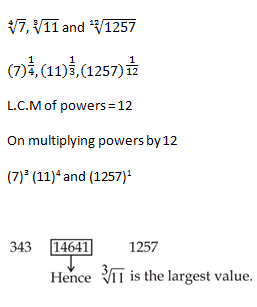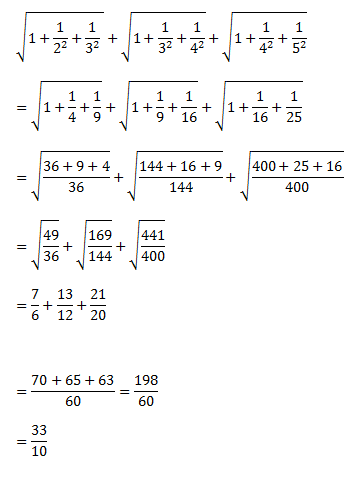(203 + 107)² – (203 – 107)²

We know (a + b)² – (a – b)² = 4ab

= 4 × 203 × 107 = 86884

By putting values from options one by one, only 468 divided by 18. The value of N = 8

31² = 961

32² = 1024

So, 1024 is the smallest four-digit number this is perfect square of 32.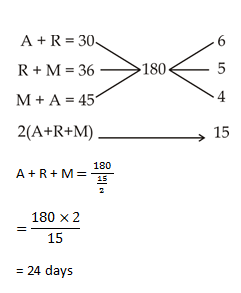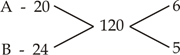Tank should be exactly filled in 12 hr

Let x is the time when A should be closed

So, A and B fill the tank for x hrs = 11x

And B alone fill the tank for (12 -x) hrs = 5(12 – x)

Now,

120 = 11x + 5 (12 – x)

120 = 11x + 60 – 5x

6x = 60

x= 10 hr

So, tank A should be closed at 5AM + 10hr = 3 PM

SP = 36800 ×  76/100

= 27968 Rs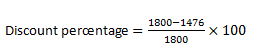= 324/18

= 18%

Let no. are 5x and 11x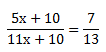65x + 130 = 77x + 70

60 = 12x

x= 5

The sum of two numbers = 5x + 11x

= 16x

= 16 × 5

= 80

 Check Here to View SSC CGL / CHSL 2021 Quantitative Aptitude Questions Day – 43 Day – 42 Day – 41